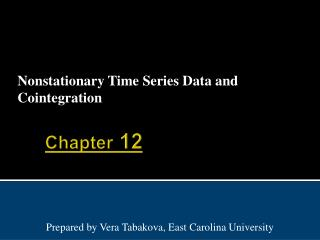DownloadDownload PresentationChapter 12

# Chapter 12

Download Presentation## Chapter 12

- - - - - - - - - - - - - - - - - - - - - - - - - - - E N D - - - - - - - - - - - - - - - - - - - - - - - - - - -
##### Presentation Transcript

1. Nonstationary Time Series Data and Cointegration Chapter 12 Prepared by Vera Tabakova, East Carolina University

2. Chapter 12: Nonstationary Time Series Data and Cointegration • 12.1 Stationary and Nonstationary Variables • 12.2 Spurious Regressions • 12.3 Unit Root Tests for Stationarity • 12.4 Cointegration • 12.5 Regression When There is No Cointegration Principles of Econometrics, 3rd Edition

3. 12.1 Stationary and Nonstationary Variables Figure 12.1(a) US economic time series Principles of Econometrics, 3rd Edition

4. 12.1 Stationary and Nonstationary Variables Figure 12.1(b) US economic time series Principles of Econometrics, 3rd Edition

5. 12.1 Stationary and Nonstationary Variables Principles of Econometrics, 3rd Edition

6. 12.1 Stationary and Nonstationary Variables Principles of Econometrics, 3rd Edition

7. 12.1.1 The First-Order Autoregressive Model Principles of Econometrics, 3rd Edition

8. 12.1.1 The First-Order Autoregressive Model Principles of Econometrics, 3rd Edition

9. 12.1.1 The First-Order Autoregressive Model Principles of Econometrics, 3rd Edition

10. 12.1.1 The First-Order Autoregressive Model Figure 12.2 (a) Time Series Models Principles of Econometrics, 3rd Edition

11. 12.1.1 The First-Order Autoregressive Model Figure 12.2 (b) Time Series Models Principles of Econometrics, 3rd Edition

12. 12.1.1 The First-Order Autoregressive Model Figure 12.2 (c) Time Series Models Principles of Econometrics, 3rd Edition

13. 12.1.2 Random Walk Models Principles of Econometrics, 3rd Edition

14. 12.1.2 Random Walk Models Principles of Econometrics, 3rd Edition

15. 12.1.2 Random Walk Models Principles of Econometrics, 3rd Edition

16. 12.1.2 Random Walk Models Principles of Econometrics, 3rd Edition

17. 12.1.2 Random Walk Models Principles of Econometrics, 3rd Edition

18. 12.2 Spurious Regressions Principles of Econometrics, 3rd Edition

19. 12.2 Spurious Regressions Figure 12.3 (a) Time Series of Two Random Walk Variables Principles of Econometrics, 3rd Edition

20. 12.2 Spurious Regressions Figure 12.3 (b) Scatter Plot of Two Random Walk Variables Principles of Econometrics, 3rd Edition

21. 12.3 Unit Root Test for Stationarity • 12.3.1 Dickey-Fuller Test 1 (no constant and no trend) Principles of Econometrics, 3rd Edition

22. 12.3 Unit Root Test for Stationarity • 12.3.1 Dickey-Fuller Test 1 (no constant and no trend) Principles of Econometrics, 3rd Edition

23. 12.3 Unit Root Test for Stationarity • 12.3.2 Dickey-Fuller Test 2 (with constant but no trend) Principles of Econometrics, 3rd Edition

24. 12.3 Unit Root Test for Stationarity • 12.3.3 Dickey-Fuller Test 3 (with constant and with trend) Principles of Econometrics, 3rd Edition

25. 12.3.4 The Dickey-Fuller Testing Procedure First step: plot the time series of the original observations on the variable. • If the series appears to be wandering or fluctuating around a sample average of zero, use test equation (12.5a). • If the series appears to be wandering or fluctuating around a sample average which is non-zero, use test equation (12.5b). • If the series appears to be wandering or fluctuating around a linear trend, use test equation (12.5c). Principles of Econometrics, 3rd Edition

26. 12.3.4 The Dickey-Fuller Testing Procedure Principles of Econometrics, 3rd Edition

27. 12.3.4 The Dickey-Fuller Testing Procedure • An important extension of the Dickey-Fuller test allows for the possibility that the error term is autocorrelated. • The unit root tests based on (12.6) and its variants (intercept excluded or trend included) are referred to as augmented Dickey-Fuller tests. Principles of Econometrics, 3rd Edition

28. 12.3.5 The Dickey-Fuller Tests: An Example Principles of Econometrics, 3rd Edition

29. 12.3.6 Order of Integration Principles of Econometrics, 3rd Edition

30. 12.4 Cointegration Principles of Econometrics, 3rd Edition

31. 12.4 Cointegration Principles of Econometrics, 3rd Edition

32. 12.4.1 An Example of a Cointegration Test Principles of Econometrics, 3rd Edition

33. 12.4.1 An Example of a Cointegration Test The null and alternative hypotheses in the test for cointegration are: Principles of Econometrics, 3rd Edition

34. 12.5 Regression When There Is No Cointegration • 12.5.1 First Difference Stationary The variable yt is said to be a first difference stationary series. Principles of Econometrics, 3rd Edition

35. 12.5.1 First Difference Stationary Principles of Econometrics, 3rd Edition

36. 12.5.2 Trend Stationary where and Principles of Econometrics, 3rd Edition

37. 12.5.2 Trend Stationary To summarize: • If variables are stationary, or I(1) and cointegrated, we can estimate a regression relationship between the levels of those variables without fear of encountering a spurious regression. • If the variables are I(1) and not cointegrated, we need to estimate a relationship in first differences, with or without the constant term. • If they are trend stationary, we can either de-trend the series first and then perform regression analysis with the stationary (de-trended) variables or, alternatively, estimate a regression relationship that includes a trend variable. The latter alternative is typically applied. Principles of Econometrics, 3rd Edition

38. Keywords • Augmented Dickey-Fuller test • Autoregressive process • Cointegration • Dickey-Fuller tests • Mean reversion • Order of integration • Random walk process • Random walk with drift • Spurious regressions • Stationary and nonstationary • Stochastic process • Stochastic trend • Tau statistic • Trend and difference stationary • Unit root tests Principles of Econometrics, 3rd Edition